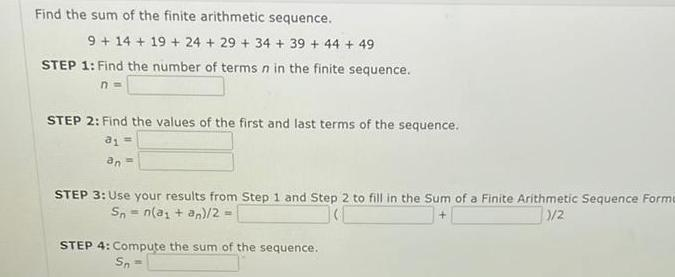Question:

# Find the sum of the finite arithmetic sequence 9 14 19 24 29

Last updated: 11/17/2023Find the sum of the finite arithmetic sequence 9 14 19 24 29 34 39 44 49 STEP 1 Find the number of terms n in the finite sequence n STEP 2 Find the values of the first and last terms of the sequence 81 an STEP 3 Use your results from Step 1 and Step 2 to fill in the Sum of a Finite Arithmetic Sequence Forme 1 2 Sn n a an 2 STEP 4 Compute the sum of the sequence Sn